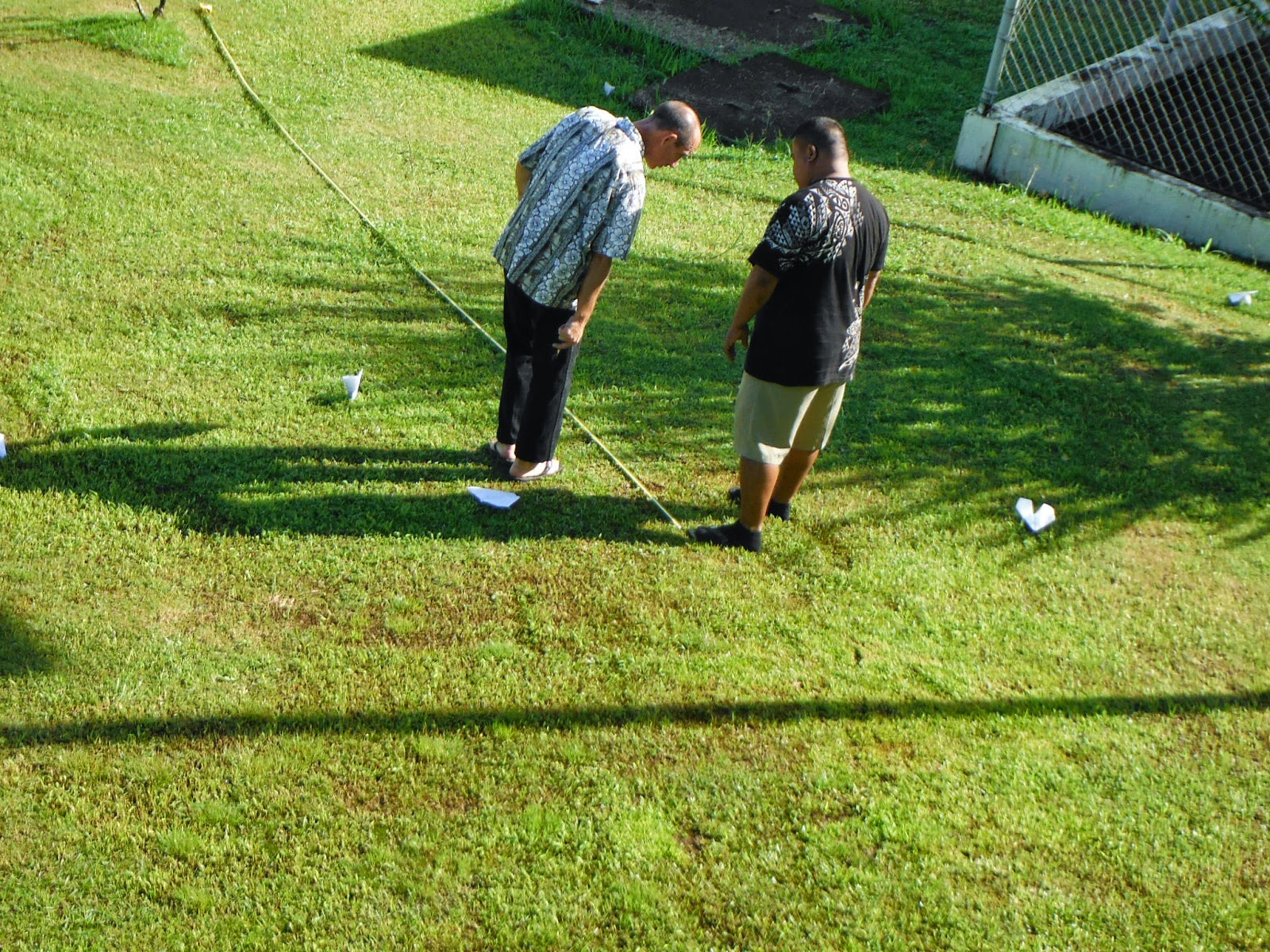### Assessing learning in introductory statistics

MS 150 Statistics has for the past three years utilized a modified curriculum based on a proposed outline. The three course level student learning outcomes for MS 150 Statistics are:

1. Perform basic statistical calculations for a single variable up to and including graphical analysis, confidence intervals, hypothesis testing against an expected value, and testing two samples for a difference of means.
2. Perform basic statistical calculations for paired correlated variables.
3. Engage in data exploration and analysis using appropriate statistical techniques including numeric calculations, graphical approaches, and tests.

The first two outcomes involve basic calculation capabilities of the students and are assessed via an item analysis of the final examination. 76 students in three sections took the final examination.Measuring flight distance towards calculating a 95% confidence interval for the flight distances. Students build and throw their own aircraft, then the calculation shows that the confidence interval captures a previously published population mean distance.
Twenty-one questions on the final examination required the students to perform basic statistical calculations on a small sample. Based on the item analysis, 80.2% of the items were answered correctly by the students. In general basic single variable statistical calcuations are an area of strength for the students.

Performance on the second student learning outcome was measured by eight questions on the final examination. Student performance on this section was lower at 68.2%. This section has historically been weaker than the basic single variable statistics section.

The third student learning outcome, open data analysis, was assessed using a simple rubric that looked at whether a student made an appropriate analysis with correct answers to questions posed in the problem and the level of statistical support for those answers. The results for the 76 students are reported in the following table and graphically in the chart.

 n RF Performance 10 0.132 An appropriate analysis with optimal statistical support for that analysis 20 0.263 An appropriate analysis with reasonable statistical support for that analysis 9 0.118 An appropriate analysis with minimal statistical support for that analysis 18 0.237 Specific questions are answered correctly but without statistical support 17 0.224 An inappropriate analysis with incorrect answers to posed questions 2 0.026 A statistical analysis that should have led to correct answers to posed questions, but those questions were left unanswered.

51% of the students answered correctly with varying levels of appropriate statistical support. Another 24% were able to obtain correct answers but did not cite supporting statistical evidence. Just under a quarter of the students, 22%, answered incorrectly. Some of the incorrect answers included the appropriate statistical analysis, but the wrong conclusion was drawn from the results. Other incorrect answers were when the student left the section blank. Observations during the examination did not indicate that students "ran out of time" to work this section, but rather simply did not know what to do with the open data exploration.

Overall performance on this section on a point basis was weak with a 36.5% average on this material - only optimal answers received full credit in points.

Data rarely comes wrapped up with nice neat specific statistical calculation questions such as "What is the mean of this data?" Data comes with general questions and the data analyst has to choose the appropriate tools for the analysis. The open data exploration seeks to probe the students' ability to handle data in the "wild."

The three sections corresponding to the three student learning outcomes have been measured since the fall of 2012. The following chart provides some historical perspective on these values.

The chart shows student success rate performance against the three proposed outcomes for the past five terms. The uppermost, yellow, circles are performance levels on single variable basic statistical calculations - course learning outcome number one. The middle, blue, circles are performance levels on two variable linear regression calculations - course learning outcome number two. The lowermost, orange, circles are the performance on the open data exploration. Note that the variation in performance on the open data exploration is due in part to differences in the marking schemes term-on-term. To some extent the open data exploration is not comparable across terms due to differences in the marking schemes. The marking scheme used fall 2014 is similar to that used spring 2014 and those performances can be compared.

Overall students prove quite capable, by term end, of making specific statistical calculations when told what calculation to make. When given data and questions that do not explain what statistics should be calculated, student performance is weaker. This addition of open data exploration to the course was stimulated by the American Statistical Association's Guidelines for Assessment and Instruction in Statistics Education. In the full report the ASA recommends to "give students plenty of practice with choosing appropriate questions and techniques, rather than telling them which technique
to use and merely having them implement it." The ASA also calls on statistics instructors to move from using naked or realistic data to using real data. My data remains in the realistic realm more than in the real realm, the exercise does require the student to choose the appropriate techniques. MS 150 Statistics continues to seek to implement best practices in the field of statistical education.